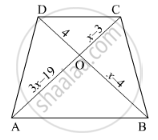Share

Books Shortlist
Your shortlist is empty

# Solution for In Below Figure, Ab || Cd. If Oa = 3x – 19, Ob = X – 4, Oc = X – 3 and Od = 4, Find X. - CBSE Class 10 - Mathematics

#### Question

In below figure, AB || CD. If OA = 3x – 19, OB = x – 4, OC = x – 3 and OD = 4, find x.#### Solution

Since diagonals of a trapezium divide each other proportionally.

therefore"AO"/"OC"="BO"/"OD"

rArr(3x-19)/(x-3)=(x-4)/4

⇒ 4(3𝑥 − 19) = (𝑥 − 4)(𝑥 − 3)

⇒ 12x – 76 = x (x – 3) −4(x – 3)

⇒ 12𝑥 − 76 = 𝑥2 − 3𝑥 − 4𝑥 + 12

⇒ 𝑥2 − 7𝑥 − 12𝑥 + 12 + 76 = 0

⇒ 𝑥2 − 19𝑥 + 88 = 0

⇒ 𝑥2 − 11x − 8x + 88 = 0

⇒ 𝑥(𝑥 − 11) − 8(𝑥 − 11) = 0

⇒ (𝑥 − 11)(𝑥 − 8) = 0

⇒ 𝑥 − 11 = 0 or x – 8 = 0

⇒ x = 11 or x = 8

Is there an error in this question or solution?

#### Video TutorialsVIEW ALL 

Solution for question: In Below Figure, Ab || Cd. If Oa = 3x – 19, Ob = X – 4, Oc = X – 3 and Od = 4, Find X. concept: Triangles Examples and Solutions. For the course CBSE
S